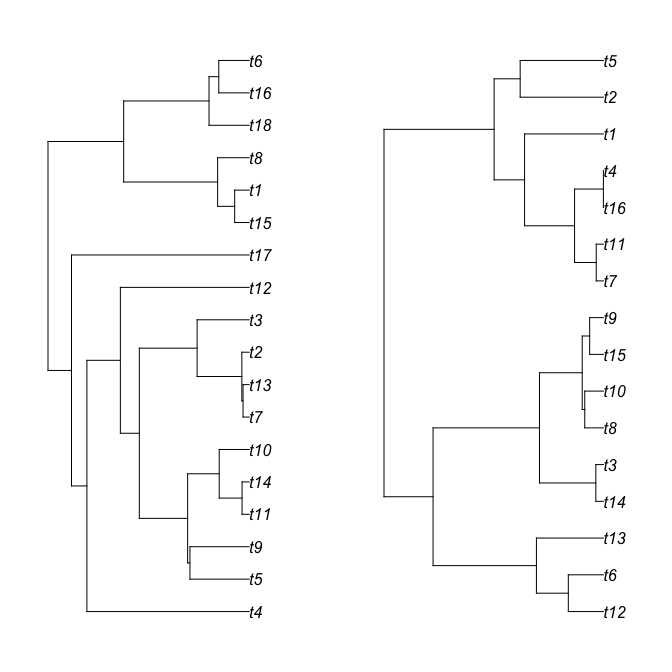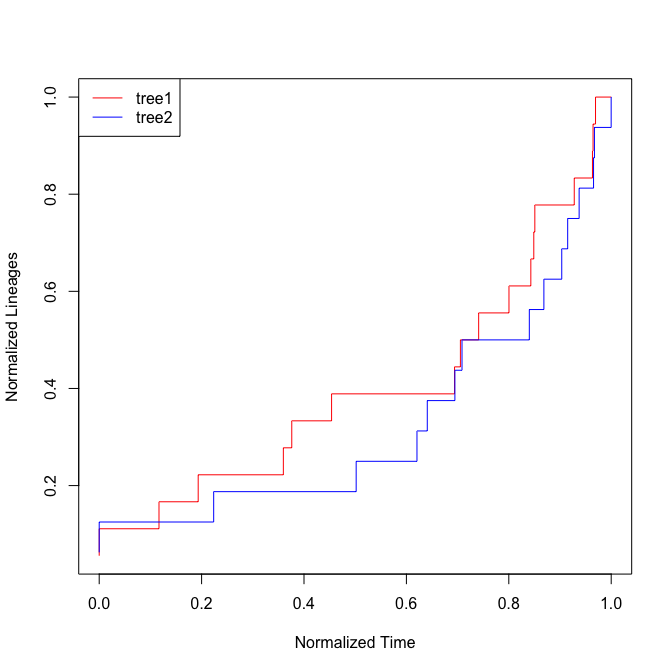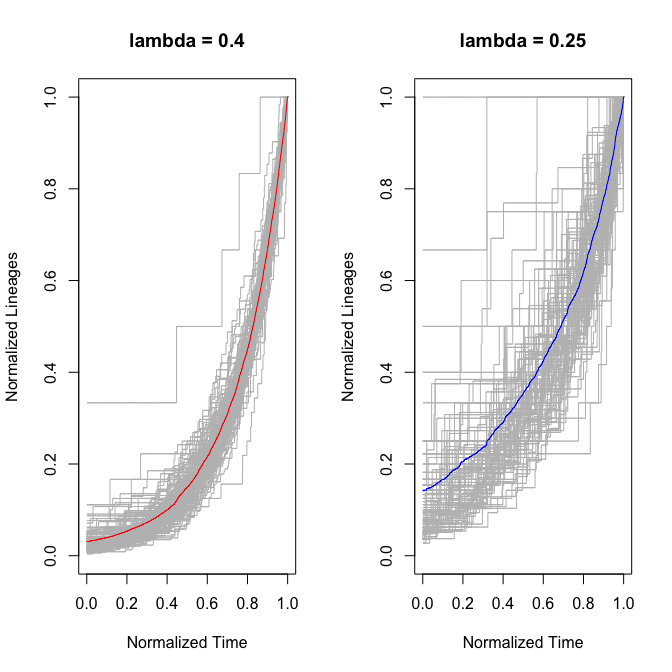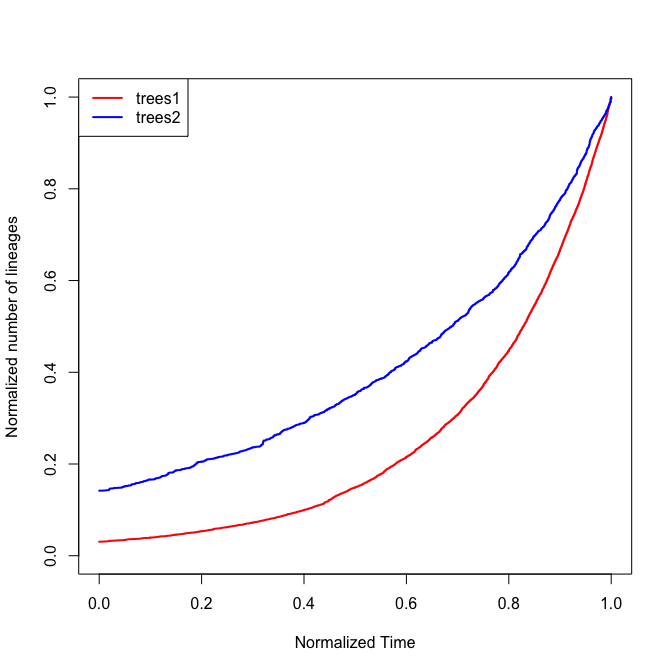# Walkthrough using the nLTT package

## nLTT statistic & nLTT plot

In order to provide some worked examples, we first generate two random phylogenetic trees, with similar parameter settings. Using the TESS package we simulate one tree with lambda = 0.4, and one tree with lambda = 0.25, both for 10 million years.

    library(nLTT) #nolint
set.seed(42)
tree1 <- TESS::tess.sim.age(n = 1, age = 10, lambda = 0.4, mu = 0)[]
tree2 <- TESS::tess.sim.age(n = 1, age = 10, lambda = 0.25, mu = 0)[]
par(mfrow = c(1, 2))
par(mar = c(2, 2, 2, 2))
plot(tree1)
plot(tree2)The two trees look similar, but are not identical in shape.

This becomes even more obvious when we plot their normalized Lineage Through Time plots:

    nltt_plot(tree1, col = "red")
nltt_lines(tree2, col = "blue")
legend("topleft", c("tree1", "tree2"), col = c("red", "blue"), lty = 1)Furthermore, we can now calculate the difference between the two nLTT lines using nLTTstat, and its more precise counterpart nLTTstat_exact:

nLTTstat(tree1, tree2) #nolint
##  0.07697467
nLTTstat_exact(tree1, tree2) #nolint
##  0.07697467

Which yields almost identical results. The function nLTTstat constructs two approximate step functions, from which it calculates the difference and integrates this difference from 0 to 1. The function nLTTstat_exact iterates through all the (normalized) branching times and for each time point calculates the difference and multiplies it with the time since the previous timepoint (e.g. manual integration). Typically results for nLTTstat and nLTTstat_exact are highly similar. For smaller trees nLTTstat_exact is preferred, as it has higher accuracy, and is faster. For extremely large trees (+500 tips) nLTTstat is faster, and the sacrifice in accuracy is minimal.

## Average nLTT matrix & Average nLTT plot

Sometimes, it might be more interesting to look at the average nLTT plot across a large number of replicate trees, or to calculate the average normalized Lineages Through Time, for a large number of replicate trees.

Let us first generate 100 random trees:

set.seed(42)
trees1 <- TESS::tess.sim.age(n = 100, age = 10, lambda = 0.4, mu = 0)
trees2 <- TESS::tess.sim.age(n = 100, age = 10, lambda = 0.25, mu = 0)

Using the function nltts_plot we can now plot the normalized Lineages Through Time for all replicates, and on top of that plot the average normalized Lineages Through Time. The replicates are all plotted in grey, and the average lines are plotted in red and blue respectively:

par(mfrow = c(1, 2))
nltts_plot(trees1, dt = 0.001, plot_nltts = TRUE,
col = "red", main = "lambda = 0.4")
nltts_plot(trees2, dt = 0.001, plot_nltts = TRUE,
col = "blue", main = "lambda = 0.25")Instead of plotting the average normalized Lineages Through Time, we can also calculate them, and store them in a matrix:

m1 <- get_average_nltt_matrix(trees1, dt = 0.001)
m2 <- get_average_nltt_matrix(trees2, dt = 0.001)

This allows us to do further analysis, and also allows us to plot the two average nLTT plots on top of each other, to see whether the two average nLTT plots differ from each other (mind you, m1 and m2 are matrices, not nLTT objects, so we can plot them using the normal plotting tools available in R)

m1 <- get_average_nltt_matrix(trees1, dt = 0.001)
m2 <- get_average_nltt_matrix(trees2, dt = 0.001)
plot(m1, type = "s", col = "red", lwd = 2, xlim = c(0, 1), ylim = c(0, 1),
xlab = "Normalized Time", ylab = "Normalized number of lineages")
lines(m2, type = "s", col = "blue", lwd = 2)
legend("topleft", c("trees1", "trees2"), col = c("red", "blue"),
lty = 1, lwd = 2)## Using nLTT within an ABC-SMC framework

In our paper introducing the nLTT statistic, we have shown how the nLTT can be used instead of the traditional likelihood, especially for situations in which the likelihood is not available. How would this work in practice?

Firstly, we have to define some prerequisites. We need a function that simulates a phylogenetic tree, given parameters. We need a prior generating distribution and a prior density function.

fixed_issue <- FALSE
 treesim <- function(params) {
t <- TreeSim::sim.bd.taxa.age(n = 100,
numbsim = 1,
lambda = params,
mu = 0, age = 10)[]
return(t)
}

As simulation function we make use of the TESS package again, this time simulating trees conditional on the number of taxa. For the sake of simplicity, we only fit lambda, and keep mu on 0.

  prior_gen <- function() {
rexp(n = 1, rate = 10)
}

prior_dens <- function(val) {
dexp(val, rate = 10)
}

statwrapper <- function(tree1) {
nLTTstat_exact(tree1, obs, "abs")  #nolint
}

As prior generating function, we choose an exponential distribution with mean 0.1. Similarly, as prior density function we choose the same distribution. Lastly, we define a function that takes a tree as argument, and compares it with the observed tree. Indeed this means that we will have to define our observed tree as a starting point:

set.seed(42)
obs <- treesim(c(0.50, 0)) #lambda = 0.5, mu = 0.0

We are now all set to do our ABC-SMC analysis! We choose a small number of particles for the sake of example, for higher accuracy one should pick a higher number of particles (at the cost of increased computation time). Similarly, better results can be obtained using a lower stop rate. We let the epsilon values decrease exponentially with each iteration of the ABC-SMC, with starting value of 0.2.

if (fixed_issue) {
a <- abc_smc_nltt(
obs, c(statwrapper), treesim, init_epsilon_values = 0.2,
prior_generating_function = prior_gen,
prior_density_function = prior_dens,
number_of_particles = 100, sigma = 0.05, stop_rate = 0.01)
}

The ABC-SMC algorithm runs until the 7th iteration. At the 7th iteration, the acceptance rate has dropped below 0.01, and the algorithm stops. We can visualize our output:

if (fixed_issue) {
hist(A, breaks = seq(0, 1, by = 0.05), col = "grey", main = "Lambda")
abline(v = 0.5, lty = 2, col = "blue", lwd = 2)
}

We see that although the peak of the posterior distribution does not lie on the simulated value (blue dotted line), generally the distribution does encompass the simulated value well. Perhaps using more particles would’ve given a better estimate. The nLTT package also contains functions to compare performance of the nLTT statistic with that of traditional likelihood methods. Let us first explore a likelihood function:

ll_b <- function(params, phy) {
lnl <- TESS::tess.likelihood(ape::branching.times(phy),
lambda = params, mu = 0.0,
samplingProbability = 1, log = TRUE)
prior1  <- log(prior_dens(params)) # nolint
return(lnl + prior1)
}

We can now first, to get a general idea, use maximum likelihood in order to find the value of lambda for which the likelihood is maximized:

if (fixed_issue) {
fun <- function(x) {
return(-1 * ll_b(x, obs)) # nolint
}
ml <- stats::optimize(f = fun, interval = c(0, 1))
ml
hist(a, breaks = seq(0, 1, by = 0.05), col = "grey", main = "Lambda")
abline(v = 0.5, lty = 2, col = "blue", lwd = 2)
abline(v = ml$minimum, lty = 2, col = "green", lwd = 2) legend("topright", c("ABC-SMC", "ML", "True"), pch = c(15, NA, NA), lty = c(NA, 2, 2), col = c("grey", "green", "blue"), lwd = 2) } The maximum likelihood method shows that the actual maximum of the tree is a bit lower than the value that we simulated with (0.42), which in turn is exactly where the highest posterior density of our ABC method is! Awesome isn’t it? Maximum likelihood, although a neat comparison, is a bit of an unfair comparison as it does not use the Bayesian framework, as the ABC-SMC method does. Alternatively, we can therefore also use a Metropolis-Hastings MCMC framework to estimate the posterior density, given a likelihood. The nLTT package provides a readily made function for that as well. if (fixed_issue) { b <- mcmc_nltt(obs, ll_b, parameters = c(0.5), logtransforms = c(TRUE), iterations = 10000, burnin = 1000, thinning = 1, sigma = 1) } We have to provide the initialvalue (parameters = 0.5), set a flag whether we want to make jumps in log space, to avoid values below zero (logtransforms = c(TRUE)), and we set a limited number of iterations, in order to avoid long computation times. Lastly, we define burnin as 10% of the chain, do not thin the chain, and set the jumping distance relatively large at 1. The MCMC chain appears to have performed well, the chain shows good mixing and doesn’t get stuck in local minima. if (fixed_issue) { b_mcmc <- coda::as.mcmc(b) plot(b_mcmc) } When we compare estimates obtained using the nLTT statistic within an ABC framework, and estimates obtained using the traditional likelihood within an MCMC framework, we find that both methods perform on par. Both methods recover the parameter value with highest likelihood, which in turn is not necessarily the true value. if (fixed_issue) { par(mfrow = c(1, 2)) hist(a, breaks = seq(0, 1, by = 0.05), col = "grey", main = "Lambda, ABC", xlab = "") abline(v = 0.5, lty = 2, col = "blue", lwd = 2) abline(v = ml$minimum, lty = 2, col = "green", lwd = 2)
legend("right", c("ML", "True"),
lty = c(2, 2),
col = c("green", "blue"), lwd = 2)
hist(b, breaks = seq(0, 1, by = 0.05), col = "grey",
main = "Lambda, MCMC", xlab = "")
abline(v = 0.5, lty = 2, col = "blue", lwd = 2)
abline(v = ml\$minimum, lty = 2, col = "green", lwd = 2)
}